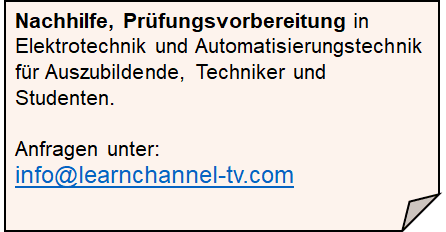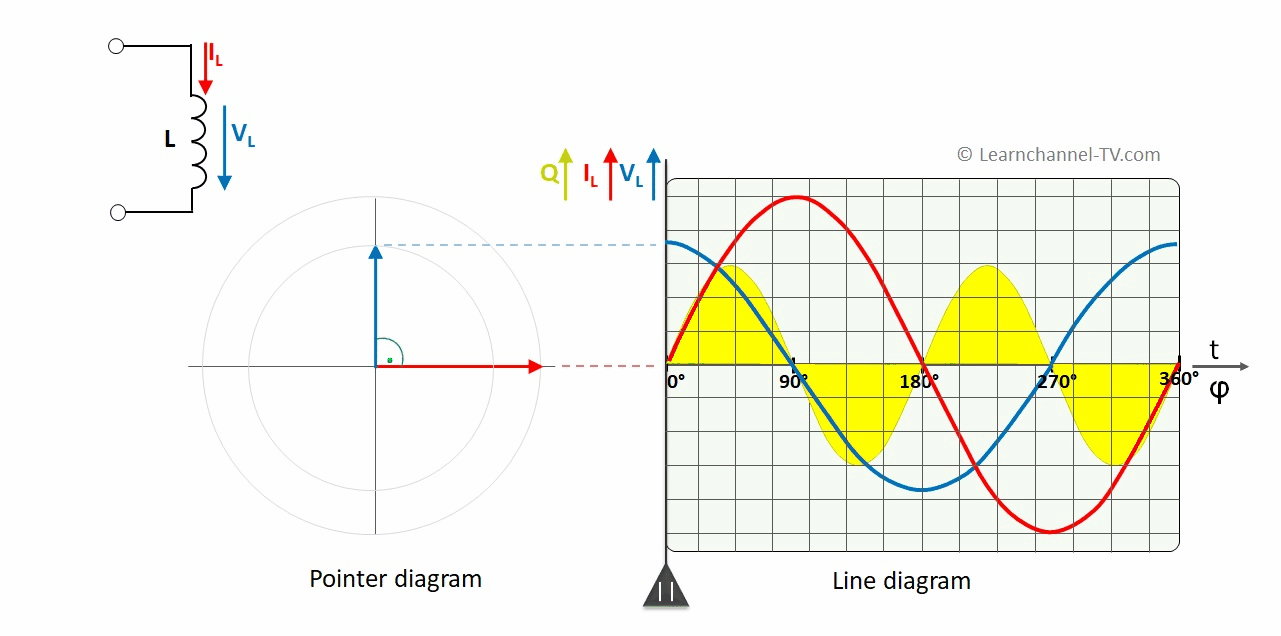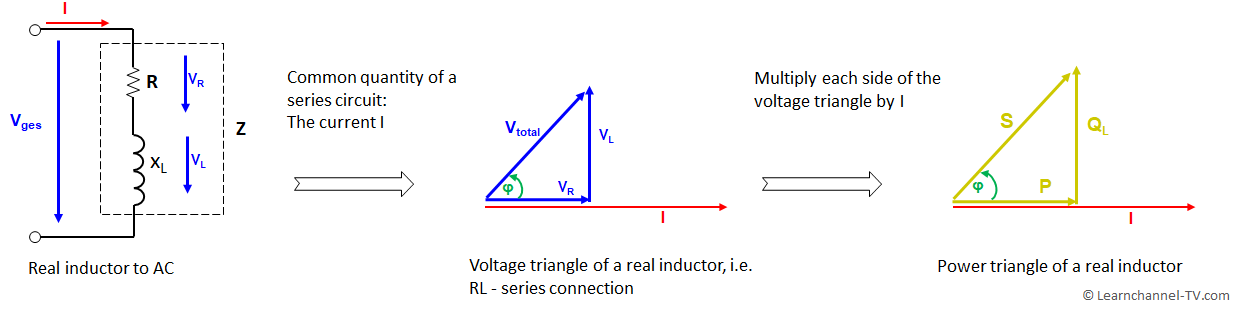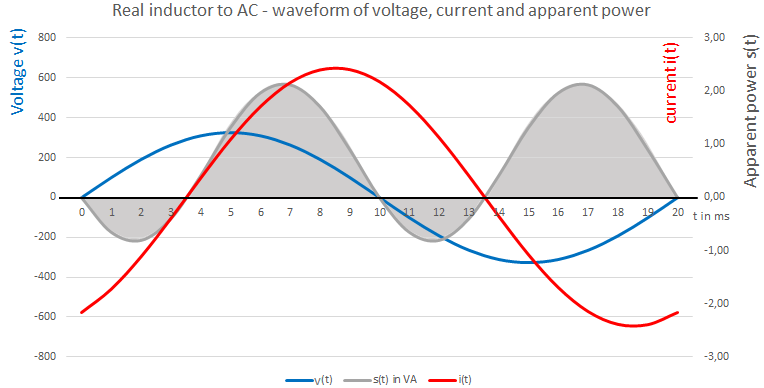# Active power, Reactive Power, Apparent Power

(Deutsch)Learnchannel Nachhilfe

### Active power, Reactive Power, Apparent Power

The ideal inductor has no wire resistance, i.e. ohmic resistance. Here the phase shift between current and voltage is 90° with the voltage leading by 90 degrees.Ideal Inductor to AC - Phase shift between voltage and current

If you determine the power as a product of voltage and current, you can see in the line diagram, that the positive and negative areas are the same size. This energy oscillates back and forth between consumer and generator and is therefore referred to as reactive power.

This reactive power, which cannot be converted into work, has its own formula symbol and unit:

[Qbl] = VAr in words: volt-ampere reactive

For the real world inductor, the wire resistance is taken into account. In the equivalent circuit, the ohmic resistance, i.e. the wire resistance, is in series with the inductive resistance:Real world inductor to AC and its equivalent circuit diagram

It is now clear that with the real inductor, in addition to a reactive power, we also have an active power due to the ohmic resistance. The total power, which is called apparent power, can be derived from the voltage triangle and results from Pythagoras:Reactive Power, Apparent Power, Active Power of a real world inductor - Power Triangle

If we consider the real inductor as a RL series circuit, we can draw a pointer diagram of the voltages. The current forms the common electrical quantity, as in any series circuit. We may multiply each side of this voltage triangle by the current I and get to a concruent, i.e. equal-angle power triangle.

As we see, this apparent power S is composed of active power and reactive power and has its own formula symbol and unit:

[S] = VA   in words: Volt-Ampere

How a possible waveform of voltage, current and apparent power looks like is shown in the following line diagram:Real inductor to AC - waveform of voltage, current and apparent power

For easy explanation, all the related quantities like Real Power, Reactive Power and Apparent Power can be easily understand by the funny Beer Analogy.Real Power, Reactive Power and Apparent Power - funny Beer Analogy